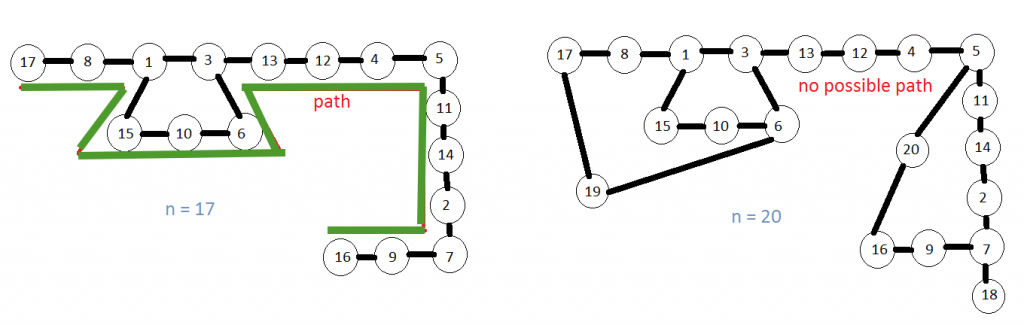Related Articles

# Permutation of numbers such that sum of two consecutive numbers is a perfect square

• Difficulty Level : Hard
• Last Updated : 27 Nov, 2018

Prerequisite: Hamiltonian Cycle

Given an integer n(>=2), find a permutation of numbers from 1 to n such that the sum of two consecutive numbers of that permutation is a perfect square. If that kind of permutation is not possible to print “No Solution”.

Examples:

```Input : 17
Output : [16, 9, 7, 2, 14, 11, 5, 4, 12, 13, 3, 6, 10, 15, 1, 8, 17]
Explanation : 16+9 = 25 = 5*5, 9+7 = 16 = 4*4, 7+2 = 9 = 3*3 and so on.

Input: 20
Output: No Solution

Input : 25
Output : [2, 23, 13, 12, 24, 25, 11, 14, 22, 3, 1, 8,
17, 19, 6, 10, 15, 21, 4, 5, 20, 16, 9, 7, 18]
```

## Recommended: Please try your approach on {IDE} first, before moving on to the solution.

Method:
We can represent a graph, where numbers from 1 to n are the nodes of the graph and there is an edge between ith and jth node if (i+j) is a perfect square. Then we can search if there is any Hamiltonian Path in the graph. If there is at least one path then we print a path otherwise we print “No Solution”.Approach:

```1. First list up all the perfect square numbers
which we can get by adding two numbers.
We can get at max (2*n-1). so we will take
only the squares up to (2*n-1).
2. Take an adjacency matrix to represent the graph.
3. For each number from 1 to n find out numbers with
which it can add upto a perfect square number.
Fill respective cells of the adjacency matrix by 1.
4. Now find if there is any Hamiltonian path in the
graph using backtracking as discussed earlier.
```

## Python3

 `# Python3 program for Sum-square series using``# hamiltonian path concept and backtracking`` ` `# Function to check wheter we can add number``# v with the path in the position pos.``def` `issafe(v, graph, path, pos):``     ` `    ``# if there is no edge between v and the``    ``# last element of the path formed so far``    ``# return false.``    ``if` `(graph[path[pos ``-` `1``]][v] ``=``=` `0``):``        ``return` `False``     ` `    ``# Otherwise if there is an edge between ``    ``# v and last element of the path formed so``    ``# far, then check all the elements of the``    ``# path. If v is already in the path return``    ``# false.``    ``for` `i ``in` `range``(pos):``         ` `        ``if` `(path[i] ``=``=` `v):``            ``return` `False`` ` `    ``# If none of the previous cases satisfies``    ``# then we can add v to the path in the``    ``# position pos. Hence return true.``    ``return` `True`` ` `# Function to form a path based on the graph.``def` `formpath(graph, path, pos):`` ` `    ``# If all the elements are included in the``    ``# path i.e. length of the path is n then``    ``# return true i.e. path formed.``    ``n ``=` `len``(graph) ``-` `1``    ``if` `(pos ``=``=` `n ``+` `1``):``        ``return` `True`` ` `    ``# This loop checks for each element if it``    ``# can be fitted as the next element of the``    ``# path and recursively finds the next ``    ``# element of the path.``    ``for` `v ``in` `range``(``1``, n ``+` `1``):``         ` `        ``if` `issafe(v, graph, path, pos):``            ``path[pos] ``=` `v``             ` `            ``# Recurs for next element of the path.``            ``if` `(formpath(graph, path, pos ``+` `1``) ``=``=` `True``):``                ``return` `True``             ` `            ``# If adding v does not give a solution``            ``# then remove it from path``            ``path[pos] ``=` `-``1``             ` `    ``# if any vertex cannot be added with the ``    ``# formed path then return false and ``    ``# backtracks.``    ``return` `False`` ` `# Function to find out sum-square series.``def` `hampath(n):``     ` `    ``# base case: if n = 1 there is no solution``    ``if` `n ``=``=` `1``:``        ``return` `'No Solution'`` ` `    ``# Make an array of perfect squares from 1 ``    ``# to (2 * n-1)``    ``l ``=` `list``()``     ` `    ``for` `i ``in` `range``(``1``, ``int``((``2` `*` `n``-``1``) ``*``*` `0.5``) ``+` `1``):``        ``l.append(i``*``*``2``)`` ` `    ``# Form the graph where sum of two adjacent``    ``# vertices is a perfect square``    ``graph ``=` `[[``0` `for` `i ``in` `range``(n ``+` `1``)] ``for` `j ``in` `range``(n ``+` `1``)]``     ` `    ``for` `i ``in` `range``(``1``, n ``+` `1``):``        ``for` `ele ``in` `l:``             ` `            ``if` `((ele``-``i) > ``0` `and` `(ele``-``i) <``=` `n``                ``and` `(``2` `*` `i !``=` `ele)):``                ``graph[i][ele ``-` `i] ``=` `1``                ``graph[ele ``-` `i][i] ``=` `1`` ` `    ``# strating from 1 upto n check for each ``    ``# element i if any path can be formed ``    ``# after taking i as the first element.``    ``for` `j ``in` `range``(``1``, n ``+` `1``):``        ``path ``=` `[``-``1` `for` `k ``in` `range``(n ``+` `1``)]``        ``path[``1``] ``=` `j`` ` `        ``# If starting from j we can form any path``        ``# then we will return the path``        ``if` `formpath(graph, path, ``2``) ``=``=` `True``:``            ``return` `path[``1``:]``             ` `    ``# If no path can be formed at all return``    ``# no solution.``    ``return` `'No Solution'``     ` `# Driver Function``print``(``17``, ``'->'``, hampath(``17``))``print``(``20``, ``'->'``, hampath(``20``))``print``(``25``, ``'->'``, hampath(``25``))`

Output:

```17 -> [16, 9, 7, 2, 14, 11, 5, 4, 12, 13, 3, 6, 10, 15, 1, 8, 17]
20 -> No Solution
25 -> [2, 23, 13, 12, 24, 25, 11, 14, 22, 3, 1, 8, 17, 19, 6, 10,
15, 21, 4, 5, 20, 16, 9, 7, 18]
```

Discussion:
This backtracking algorithm takes exponential time to find Hamiltonian Path. Hence the time complexity of this algorithm is exponential.
In the last part of the hampath(n) function if we just print the path rather returning it then it will print all possible Hamiltonian Path i.e. all possible representations.
Actually we will first get a representation like this for n = 15. For n<15 there is no representation. For n = 18, 19, 20, 21, 22, 24 there is also no Hamiltonian Path. For rest of the numbers it works well.

Reference: Numberphile

Attention reader! Don’t stop learning now. Get hold of all the important DSA concepts with the DSA Self Paced Course at a student-friendly price and become industry ready.  To complete your preparation from learning a language to DS Algo and many more,  please refer Complete Interview Preparation Course.

In case you wish to attend live classes with experts, please refer DSA Live Classes for Working Professionals and Competitive Programming Live for Students.

My Personal Notes arrow_drop_up